Note: The other languages of the website are Google-translated. Back to English

## 如何在Excel中将数字转换为印度卢比的单词？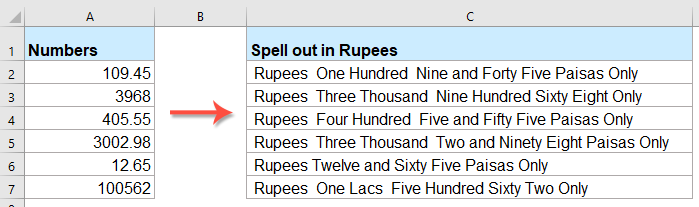#### 使用VBA代码将数字转换为印度卢比的单词

1。 按住 ALT + F11 键打开 Microsoft Visual Basic应用程序 窗口。

2。 点击 插页 > 模块，然后将以下代码粘贴到“模块窗口”中。

VBA代码：将数字转换为卢比的单词

``````Public Function RupeeFormat(SNum As String)
'Updateby Extendoffice
Dim xDPInt As Integer
Dim xArrPlace As Variant
Dim xRStr_Paisas As String
Dim xNumStr As String
Dim xF As Integer
Dim xTemp As String
Dim xStrTemp As String
Dim xRStr As String
Dim xLp As Integer
xArrPlace = Array("", "", " Thousand ", " Lacs ", " Crores ", " Trillion ", "", "", "", "")
On Error Resume Next
If SNum = "" Then
RupeeFormat = ""
Exit Function
End If
xNumStr = Trim(str(SNum))
If xNumStr = "" Then
RupeeFormat = ""
Exit Function
End If

xRStr = ""
xLp = 0
If (xNumStr > 999999999.99) Then
RupeeFormat = "Digit excced Maximum limit"
Exit Function
End If
xDPInt = InStr(xNumStr, ".")
If xDPInt > 0 Then
If (Len(xNumStr) - xDPInt) = 1 Then
xRStr_Paisas = RupeeFormat_GetT(Left(Mid(xNumStr, xDPInt + 1) & "0", 2))
ElseIf (Len(xNumStr) - xDPInt) > 1 Then
xRStr_Paisas = RupeeFormat_GetT(Left(Mid(xNumStr, xDPInt + 1), 2))
End If
xNumStr = Trim(Left(xNumStr, xDPInt - 1))
End If
xF = 1
Do While xNumStr <> ""
If (xF >= 2) Then
xTemp = Right(xNumStr, 2)
Else
If (Len(xNumStr) = 2) Then
xTemp = Right(xNumStr, 2)
ElseIf (Len(xNumStr) = 1) Then
xTemp = Right(xNumStr, 1)
Else
xTemp = Right(xNumStr, 3)
End If
End If
xStrTemp = ""
If Val(xTemp) > 99 Then
xStrTemp = RupeeFormat_GetH(Right(xTemp, 3), xLp)
If Right(Trim(xStrTemp), 3) <> "Lac" Then
xLp = xLp + 1
End If
ElseIf Val(xTemp) <= 99 And Val(xTemp) > 9 Then
xStrTemp = RupeeFormat_GetT(Right(xTemp, 2))
ElseIf Val(xTemp) < 10 Then
xStrTemp = RupeeFormat_GetD(Right(xTemp, 2))
End If
If xStrTemp <> "" Then
xRStr = xStrTemp & xArrPlace(xF) & xRStr
End If
If xF = 2 Then
If Len(xNumStr) = 1 Then
xNumStr = ""
Else
xNumStr = Left(xNumStr, Len(xNumStr) - 2)
End If
ElseIf xF = 3 Then
If Len(xNumStr) >= 3 Then
xNumStr = Left(xNumStr, Len(xNumStr) - 2)
Else
xNumStr = ""
End If
ElseIf xF = 4 Then
xNumStr = ""
Else
If Len(xNumStr) <= 2 Then
xNumStr = ""
Else
xNumStr = Left(xNumStr, Len(xNumStr) - 3)
End If
End If
xF = xF + 1
Loop
If xRStr = "" Then
xRStr = "No Rupees"
Else
xRStr = " Rupees " & xRStr
End If
If xRStr_Paisas <> "" Then
xRStr_Paisas = " and " & xRStr_Paisas & " Paisas"
End If
RupeeFormat = xRStr & xRStr_Paisas & " Only"
End Function
Function RupeeFormat_GetH(xStrH As String, xLp As Integer)
Dim xRStr As String
If Val(xStrH) < 1 Then
RupeeFormat_GetH = ""
Exit Function
Else
xStrH = Right("000" & xStrH, 3)
If Mid(xStrH, 1, 1) <> "0" Then
If (xLp > 0) Then
xRStr = RupeeFormat_GetD(Mid(xStrH, 1, 1)) & " Lac "
Else
xRStr = RupeeFormat_GetD(Mid(xStrH, 1, 1)) & " Hundred "
End If
End If
If Mid(xStrH, 2, 1) <> "0" Then
xRStr = xRStr & RupeeFormat_GetT(Mid(xStrH, 2))
Else
xRStr = xRStr & RupeeFormat_GetD(Mid(xStrH, 3))
End If
End If
RupeeFormat_GetH = xRStr
End Function
Function RupeeFormat_GetT(xTStr As String)
Dim xTArr1 As Variant
Dim xTArr2 As Variant
Dim xRStr As String
xTArr1 = Array("Ten", "Eleven", "Twelve", "Thirteen", "Fourteen", "Fifteen", "Sixteen", "Seventeen", "Eighteen", "Nineteen")
xTArr2 = Array("", "Twenty", "Thirty", "Forty", "Fifty", "Sixty", "Seventy", "Eighty", "Ninety")
Result = ""
If Val(Left(xTStr, 1)) = 1 Then
xRStr = xTArr1(Val(Mid(xTStr, 2, 1)))
Else
If Val(Left(xTStr, 1)) > 0 Then
xRStr = xTArr2(Val(Left(xTStr, 1)) - 1)
End If
xRStr = xRStr & RupeeFormat_GetD(Right(xTStr, 1))
End If
RupeeFormat_GetT = xRStr
End Function
Function RupeeFormat_GetD(xDStr As String)
Dim xArr_1() As Variant
xArr_1 = Array(" One", " Two", " Three", " Four", " Five", " Six", " Seven", " Eight", " Nine", "")
If Val(xDStr) > 0 Then
RupeeFormat_GetD = xArr_1(Val(xDStr) - 1)
Else
RupeeFormat_GetD = ""
End If
End Function
``````

3。 插入代码后，保存并关闭代码窗口，返回到工作表，然后输入以下公式： = RupeeFormat（A2） 到一个空白单元格中，然后向下拖动填充手柄以将此公式应用于其他单元格，所有数字均以卢比表示，请参见屏幕截图：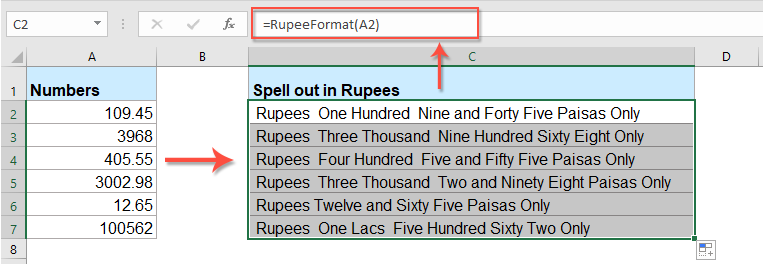#### 具有惊人功能的将数字转换为英文单词

1。 选择要转换的数字列表，然后单击 库工具 > 内容 > 数字到单词，请参见屏幕截图：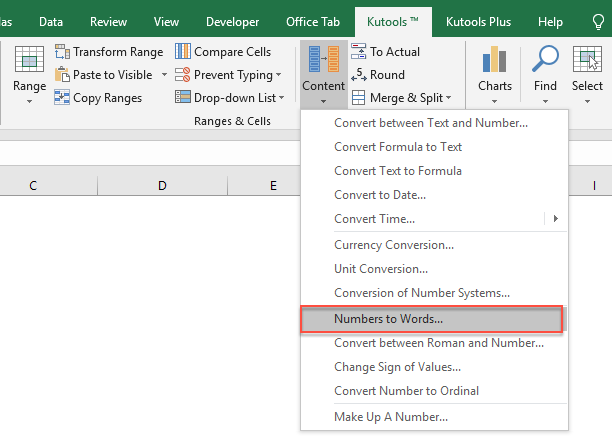2。 在 数字到货币单词 对话框中选择 英语 选项从 语言 部分，然后单击 Ok 按钮，所选内容中的数字已转换为英语货币单词，请参见屏幕截图：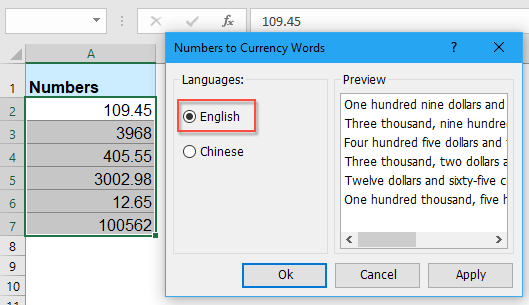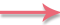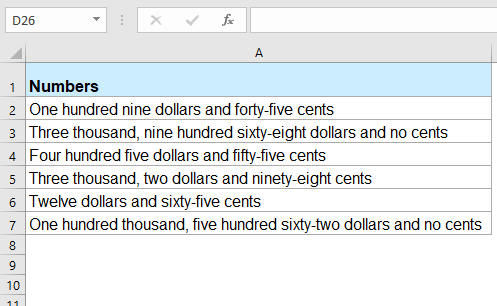## 最佳办公效率工具

### Kutools for Excel解决了您的大多数问题，并使您的生产率提高了80％

• 超级公式栏 （轻松编辑多行文本和公式）； 阅读版式 （轻松读取和编辑大量单元格）； 粘贴到过滤范围...
• 合并单元格/行/列 和保存数据； 拆分单元格内容； 合并重复的行和总和/平均值...防止细胞重复； 比较范围...
• 选择重复或唯一 行; 选择空白行 （所有单元格都是空的）； 超级查找和模糊查找 在许多工作簿中； 随机选择...
• 确切的副本 多个单元格，无需更改公式参考； 自动创建参考 到多张纸； 插入项目符号，复选框等...
• 收藏并快速插入公式，范围，图表和图片； 加密单元 带密码 创建邮件列表 并发送电子邮件...
• 提取文字，添加文本，按位置删除， 删除空间; 创建和打印分页小计； 在单元格内容和注释之间转换...
• 超级滤镜 （将过滤方案保存并应用于其他工作表）； 高级排序 按月/周/日，频率及更多； 特殊过滤器 用粗体，斜体...
• 结合工作簿和工作表; 根据关键列合并表； 将数据分割成多个工作表; 批量转换xls，xlsx和PDF...
• 数据透视表分组依据 周号，周几等 显示未锁定的单元格 用不同的颜色 突出显示具有公式/名称的单元格...### Office选项卡-将选项卡式界面引入Office，使您的工作更加轻松

• 在Word，Excel，PowerPoint中启用选项卡式编辑和阅读，发布者，Access，Visio和Project。
• 在同一窗口的新选项卡中而不是在新窗口中打开并创建多个文档。
• 每天将您的工作效率提高50％，并减少数百次鼠标单击！(10,000.99) 只有十万和 99/100 里亚尔
10,000.99 只有一万和 99/100 里亚尔
(10,000,000.99) 仅一亿和 99/100 里亚尔
10,000,000.99 仅一千万和 99/100 里亚尔
(10,000,000,000.99) 只有千百亿 & 99/100 里亚尔
10,000,000,000.99 仅 99 亿 & 100/XNUMX 里亚尔
(10,000,000,000,000.90) 仅百亿和 90/100 里亚尔
10,000,000,000,000.90 仅十万亿和 90/100 里亚尔

'主功能

ReDim Place(9) 作为字符串

Place(4) = "十亿"
Place(5) = "万亿"
' 金额的字符串表示。
MyNumber = Trim(Str(MyNumber))
' 小数点位置 0 如果没有。
DecimalPlace = InStr(MyNumber, ".")
' 转换 Halalas 并将 MyNumber 设置为里亚尔金额。

Halalas = GetTens(Left(Mid(MyNumber, DecimalPlace + 1) & _
"00", 2))
MyNumber = Trim(左(MyNumber, DecimalPlace - 1))

Do While MyNumber <> ""
Temp = GetHundreds(右(MyNumber, 3))
If Temp <> "" Then Riyals = Temp & Place(Count) & Riyals

MyNumber = Left(MyNumber, Len(MyNumber) - 3)

CASE ELSE

Halalas = " & 00/00 里亚尔"

Halalas = " & 01/100 里亚尔"

Halalas = " & 02/100 里亚尔"

Halalas = " & 03/100 里亚尔"

Halalas = " & 04/100 里亚尔"

Halalas = " & 05/100 里亚尔"

Halalas = " & 06/100 里亚尔"

Halalas = " & 07/100 里亚尔"

Halalas = " & 08/100 里亚尔"

Halalas = " & 09/100 里亚尔"

Halalas = " & 10/100 里亚尔"

Halalas = " & 11/100 里亚尔"

Halalas = " & 12/100 里亚尔"

Halalas = " & 13/100 里亚尔"

Halalas = " & 14/100 里亚尔"

Halalas = " & 15/100 里亚尔"

Halalas = " & 16/100 里亚尔"

Halalas = " & 17/100 里亚尔"

Halalas = " & 18/100 里亚尔"

Halalas = " & 19/100 里亚尔"

Halalas = " & 20/100 里亚尔"

Halalas = " & 21/100 里亚尔"

Halalas = " & 22/100 里亚尔"

Halalas = " & 23/100 里亚尔"

Halalas = " & 24/100 里亚尔"

Halalas = " & 25/100 里亚尔"

Halalas = " & 26/100 里亚尔"

Halalas = " & 27/100 里亚尔"

Halalas = " & 28/100 里亚尔"

Halalas = " & 29/100 里亚尔"

Halalas = " & 30/100 里亚尔"

Halalas = " & 31/100 里亚尔"

Halalas = " & 32/100 里亚尔"

Halalas = " & 33/100 里亚尔"

Halalas = " & 34/100 里亚尔"

Halalas = " & 35/100 里亚尔"

Halalas = " & 36/100 里亚尔"

Halalas = " & 37/100 里亚尔"

Halalas = " & 38/100 里亚尔"

Halalas = " & 39/100 里亚尔"

Halalas = " & 40/100 里亚尔"

Halalas = " & 41/100 里亚尔"

Halalas = " & 42/100 里亚尔"

Halalas = " & 43/100 里亚尔"

Halalas = " & 44/100 里亚尔"

Halalas = " & 45/100 里亚尔"

Halalas = " & 46/100 里亚尔"

Halalas = " & 47/100 里亚尔"

Halalas = " & 48/100 里亚尔"

Halalas = " & 49/100 里亚尔"

Halalas = " & 50/100 里亚尔"

Halalas = " & 51/100 里亚尔"

Halalas = " & 52/100 里亚尔"

Halalas = " & 53/100 里亚尔"

Halalas = " & 54/100 里亚尔"

Halalas = " & 55/100 里亚尔"

Halalas = " & 56/100 里亚尔"

Halalas = " & 57/100 里亚尔"

Halalas = " & 58/100 里亚尔"

Halalas = " & 59/100 里亚尔"

Halalas = " & 60/100 里亚尔"

Halalas = " & 61/100 里亚尔"

Halalas = " & 62/100 里亚尔"

Halalas = " & 63/100 里亚尔"

Halalas = " & 64/100 里亚尔"

Halalas = " & 65/100 里亚尔"

Halalas = " & 66/100 里亚尔"

Halalas = " & 67/100 里亚尔"

Halalas = " & 68/100 里亚尔"

Halalas = " & 69/100 里亚尔"

Halalas = " & 70/100 里亚尔"

Halalas = " & 71/100 里亚尔"

Halalas = " & 72/100 里亚尔"

Halalas = " & 73/100 里亚尔"

Halalas = " & 74/100 里亚尔"

Halalas = " & 75/100 里亚尔"

Halalas = " & 76/100 里亚尔"

Halalas = " & 77/100 里亚尔"

Halalas = " & 78/100 里亚尔"

Halalas = " & 79/100 里亚尔"

Halalas = " & 80/100 里亚尔"

Halalas = " & 81/100 里亚尔"

Halalas = " & 82/100 里亚尔"

Halalas = " & 83/100 里亚尔"

Halalas = " & 84/100 里亚尔"

Halalas = " & 85/100 里亚尔"

Halalas = " & 86/100 里亚尔"

Halalas = " & 87/100 里亚尔"

Halalas = " & 88/100 里亚尔"

Halalas = " & 89/100 里亚尔"

Halalas = " & 90/100 里亚尔"

Halalas = " & 91/100 里亚尔"

Halalas = " & 92/100 里亚尔"

Halalas = " & 93/100 里亚尔"

Halalas = " & 94/100 里亚尔"

Halalas = " & 95/100 里亚尔"

Halalas = " & 96/100 里亚尔"

Halalas = " & 97/100 里亚尔"

Halalas = " & 98/100 里亚尔"

Halalas = " & 99/100 里亚尔"

CASE ELSE

SpellBilling = 里亚尔和哈拉拉斯

' 将 100-999 之间的数字转换为文本

MyNumber = Right("000" & MyNumber, 3)
' 转换百位。

' 转换十位和个位。

GetHundreds = 结果

' 将 10 到 99 之间的数字转换为文本。

Result = "" ' 清空临时函数值。
If Val(Left(TensText, 1)) = 1 Then ' 如果值在 10-19 之间...

CASE ELSE

Else ' 如果值在 20-99 之间...

CASE ELSE

(Right(TensText, 1)) ' 检索一个位置。

GetTens = 结果

' 将 1 到 9 之间的数字转换为文本。

(10,000.99) 只有十万和 99/100 里亚尔
10,000.99 只有一万和 99/100 里亚尔
(10,000,000.99) 仅一亿和 99/100 里亚尔
10,000,000.99 仅一千万和 99/100 里亚尔
(10,000,000,000.99) 只有千百亿 & 99/100 里亚尔
10,000,000,000.99 仅 99 亿 & 100/XNUMX 里亚尔
(10,000,000,000,000.90) 仅百亿和 90/100 里亚尔
10,000,000,000,000.90 仅十万亿和 90/100 里亚尔

'主功能

ReDim Place(9) 作为字符串

Place(4) = "十亿"
Place(5) = "万亿"
' 金额的字符串表示。
MyNumber = Trim(Str(MyNumber))
' 小数点位置 0 如果没有。
DecimalPlace = InStr(MyNumber, ".")
' 转换 Halalas 并将 MyNumber 设置为里亚尔金额。

Halalas = GetTens(Left(Mid(MyNumber, DecimalPlace + 1) & _
"00", 2))
MyNumber = Trim(左(MyNumber, DecimalPlace - 1))

Do While MyNumber <> ""
Temp = GetHundreds(右(MyNumber, 3))
If Temp <> "" Then Riyals = Temp & Place(Count) & Riyals

MyNumber = Left(MyNumber, Len(MyNumber) - 3)

CASE ELSE

Halalas = " & 00/00 里亚尔"

Halalas = " & 01/100 里亚尔"

Halalas = " & 02/100 里亚尔"

Halalas = " & 03/100 里亚尔"

Halalas = " & 04/100 里亚尔"

Halalas = " & 05/100 里亚尔"

Halalas = " & 06/100 里亚尔"

Halalas = " & 07/100 里亚尔"

Halalas = " & 08/100 里亚尔"

Halalas = " & 09/100 里亚尔"

Halalas = " & 10/100 里亚尔"

Halalas = " & 11/100 里亚尔"

Halalas = " & 12/100 里亚尔"

Halalas = " & 13/100 里亚尔"

Halalas = " & 14/100 里亚尔"

Halalas = " & 15/100 里亚尔"

Halalas = " & 16/100 里亚尔"

Halalas = " & 17/100 里亚尔"

Halalas = " & 18/100 里亚尔"

Halalas = " & 19/100 里亚尔"

Halalas = " & 20/100 里亚尔"

Halalas = " & 21/100 里亚尔"

Halalas = " & 22/100 里亚尔"

Halalas = " & 23/100 里亚尔"

Halalas = " & 24/100 里亚尔"

Halalas = " & 25/100 里亚尔"

Halalas = " & 26/100 里亚尔"

Halalas = " & 27/100 里亚尔"

Halalas = " & 28/100 里亚尔"

Halalas = " & 29/100 里亚尔"

Halalas = " & 30/100 里亚尔"

Halalas = " & 31/100 里亚尔"

Halalas = " & 32/100 里亚尔"

Halalas = " & 33/100 里亚尔"

Halalas = " & 34/100 里亚尔"

Halalas = " & 35/100 里亚尔"

Halalas = " & 36/100 里亚尔"

Halalas = " & 37/100 里亚尔"

Halalas = " & 38/100 里亚尔"

Halalas = " & 39/100 里亚尔"

Halalas = " & 40/100 里亚尔"

Halalas = " & 41/100 里亚尔"

Halalas = " & 42/100 里亚尔"

Halalas = " & 43/100 里亚尔"

Halalas = " & 44/100 里亚尔"

Halalas = " & 45/100 里亚尔"

Halalas = " & 46/100 里亚尔"

Halalas = " & 47/100 里亚尔"

Halalas = " & 48/100 里亚尔"

Halalas = " & 49/100 里亚尔"

Halalas = " & 50/100 里亚尔"

Halalas = " & 51/100 里亚尔"

Halalas = " & 52/100 里亚尔"

Halalas = " & 53/100 里亚尔"

Halalas = " & 54/100 里亚尔"

Halalas = " & 55/100 里亚尔"

Halalas = " & 56/100 里亚尔"

Halalas = " & 57/100 里亚尔"

Halalas = " & 58/100 里亚尔"

Halalas = " & 59/100 里亚尔"

Halalas = " & 60/100 里亚尔"

Halalas = " & 61/100 里亚尔"

Halalas = " & 62/100 里亚尔"

Halalas = " & 63/100 里亚尔"

Halalas = " & 64/100 里亚尔"

Halalas = " & 65/100 里亚尔"

Halalas = " & 66/100 里亚尔"

Halalas = " & 67/100 里亚尔"

Halalas = " & 68/100 里亚尔"

Halalas = " & 69/100 里亚尔"

Halalas = " & 70/100 里亚尔"

Halalas = " & 71/100 里亚尔"

Halalas = " & 72/100 里亚尔"

Halalas = " & 73/100 里亚尔"

Halalas = " & 74/100 里亚尔"

Halalas = " & 75/100 里亚尔"

Halalas = " & 76/100 里亚尔"

Halalas = " & 77/100 里亚尔"

Halalas = " & 78/100 里亚尔"

Halalas = " & 79/100 里亚尔"

Halalas = " & 80/100 里亚尔"

Halalas = " & 81/100 里亚尔"

Halalas = " & 82/100 里亚尔"

Halalas = " & 83/100 里亚尔"

Halalas = " & 84/100 里亚尔"

Halalas = " & 85/100 里亚尔"

Halalas = " & 86/100 里亚尔"

Halalas = " & 87/100 里亚尔"

Halalas = " & 88/100 里亚尔"

Halalas = " & 89/100 里亚尔"

Halalas = " & 90/100 里亚尔"

Halalas = " & 91/100 里亚尔"

Halalas = " & 92/100 里亚尔"

Halalas = " & 93/100 里亚尔"

Halalas = " & 94/100 里亚尔"

Halalas = " & 95/100 里亚尔"

Halalas = " & 96/100 里亚尔"

Halalas = " & 97/100 里亚尔"

Halalas = " & 98/100 里亚尔"

Halalas = " & 99/100 里亚尔"

CASE ELSE

SpellBilling = 里亚尔和哈拉拉斯

' 将 100-999 之间的数字转换为文本

MyNumber = Right("000" & MyNumber, 3)
' 转换百位。

' 转换十位和个位。

GetHundreds = 结果

' 将 10 到 99 之间的数字转换为文本。

Result = "" ' 清空临时函数值。
If Val(Left(TensText, 1)) = 1 Then ' 如果值在 10-19 之间...

CASE ELSE

Else ' 如果值在 20-99 之间...

CASE ELSE

(Right(TensText, 1)) ' 检索一个位置。

GetTens = 结果

' 将 1 到 9 之间的数字转换为文本。

XNUMX Lac XNUMX 和 XNUMX Paise - 未正确转换。在四舍五入时也未转换上图。

``````Public Function RupeeFormat(SNum As String)
'Updateby Extendoffice
Dim xDPInt As Integer
Dim xArrPlace As Variant
Dim xRStr_Paisas As String
Dim xNumStr As String
Dim xF As Integer
Dim xTemp As String
Dim xStrTemp As String
Dim xRStr As String
Dim xLp As Integer
xArrPlace = Array("", "", " Thousand ", " Lacs ", " Crores ", " Trillion ", "", "", "", "")
On Error Resume Next
If SNum = "" Then
RupeeFormat = ""
Exit Function
End If
xNumStr = Trim(Str(SNum))
If xNumStr = "" Then
RupeeFormat = ""
Exit Function
End If

xRStr = ""
xLp = 0
If (xNumStr > 999999999.99) Then
RupeeFormat = "Digit excced Maximum limit"
Exit Function
End If
xDPInt = InStr(xNumStr, ".")
If xDPInt > 0 Then
If (Len(xNumStr) - xDPInt) = 1 Then
xRStr_Paisas = RupeeFormat_GetT(Left(Mid(xNumStr, xDPInt + 1) & "0", 2))
ElseIf (Len(xNumStr) - xDPInt) > 1 Then
xRStr_Paisas = RupeeFormat_GetT(Left(Mid(xNumStr, xDPInt + 1), 2))
End If
xNumStr = Trim(Left(xNumStr, xDPInt - 1))
End If
xF = 1
Do While xNumStr <> ""
If (xF >= 2) Then
xTemp = Right(xNumStr, 2)
Else
If (Len(xNumStr) = 2) Then
xTemp = Right(xNumStr, 2)
ElseIf (Len(xNumStr) = 1) Then
xTemp = Right(xNumStr, 1)
Else
xTemp = Right(xNumStr, 3)
End If
End If
xStrTemp = ""
If Val(xTemp) > 99 Then
xStrTemp = RupeeFormat_GetH(Right(xTemp, 3), xLp)
If Right(Trim(xStrTemp), 3) <> "Lac" Then
xLp = xLp + 1
End If
ElseIf Val(xTemp) <= 99 And Val(xTemp) > 9 Then
xStrTemp = RupeeFormat_GetT(Right(xTemp, 2))
ElseIf Val(xTemp) < 10 Then
xStrTemp = RupeeFormat_GetD(Right(xTemp, 2))
End If
If xStrTemp <> "" Then
xRStr = xStrTemp & xArrPlace(xF) & xRStr
End If
If xF = 2 Then
If Len(xNumStr) = 1 Then
xNumStr = ""
Else
xNumStr = Left(xNumStr, Len(xNumStr) - 2)
End If
ElseIf xF = 3 Then
If Len(xNumStr) >= 3 Then
xNumStr = Left(xNumStr, Len(xNumStr) - 2)
Else
xNumStr = ""
End If
ElseIf xF = 4 Then
xNumStr = ""
Else
If Len(xNumStr) <= 2 Then
xNumStr = ""
Else
xNumStr = Left(xNumStr, Len(xNumStr) - 3)
End If
End If
xF = xF + 1
Loop
If xRStr = "" Then
xRStr = "No Rupees"
Else
xRStr = xRStr
End If
If xRStr_Paisas <> "" Then
xRStr_Paisas = " and " & xRStr_Paisas & " Paisas"
End If
RupeeFormat = xRStr & xRStr_Paisas & " Only"
End Function
Function RupeeFormat_GetH(xStrH As String, xLp As Integer)
Dim xRStr As String
If Val(xStrH) < 1 Then
RupeeFormat_GetH = ""
Exit Function
Else
xStrH = Right("000" & xStrH, 3)
If Mid(xStrH, 1, 1) <> "0" Then
If (xLp > 0) Then
xRStr = RupeeFormat_GetD(Mid(xStrH, 1, 1)) & " Lac "
Else
xRStr = RupeeFormat_GetD(Mid(xStrH, 1, 1)) & " Hundred "
End If
End If
If Mid(xStrH, 2, 1) <> "0" Then
xRStr = xRStr & RupeeFormat_GetT(Mid(xStrH, 2))
Else
xRStr = xRStr & RupeeFormat_GetD(Mid(xStrH, 3))
End If
End If
RupeeFormat_GetH = xRStr
End Function
Function RupeeFormat_GetT(xTStr As String)
Dim xTArr1 As Variant
Dim xTArr2 As Variant
Dim xRStr As String
xTArr1 = Array("Ten", "Eleven", "Twelve", "Thirteen", "Fourteen", "Fifteen", "Sixteen", "Seventeen", "Eighteen", "Nineteen")
xTArr2 = Array("", "Twenty", "Thirty", "Forty", "Fifty", "Sixty", "Seventy", "Eighty", "Ninety")
Result = ""
If Val(Left(xTStr, 1)) = 1 Then
xRStr = xTArr1(Val(Mid(xTStr, 2, 1)))
Else
If Val(Left(xTStr, 1)) > 0 Then
xRStr = xTArr2(Val(Left(xTStr, 1)) - 1)
End If
xRStr = xRStr & RupeeFormat_GetD(Right(xTStr, 1))
End If
RupeeFormat_GetT = xRStr
End Function
Function RupeeFormat_GetD(xDStr As String)
Dim xArr_1() As Variant
xArr_1 = Array(" One", " Two", " Three", " Four", " Five", " Six", " Seven", " Eight", " Nine", "")
If Val(xDStr) > 0 Then
RupeeFormat_GetD = xArr_1(Val(xDStr) - 1)
Else
RupeeFormat_GetD = ""
End If
End Function

``````

## 关注我们

Microsoft和Office徽标是Microsoft Corporation在美国和/或其他国家的商标或注册商标。